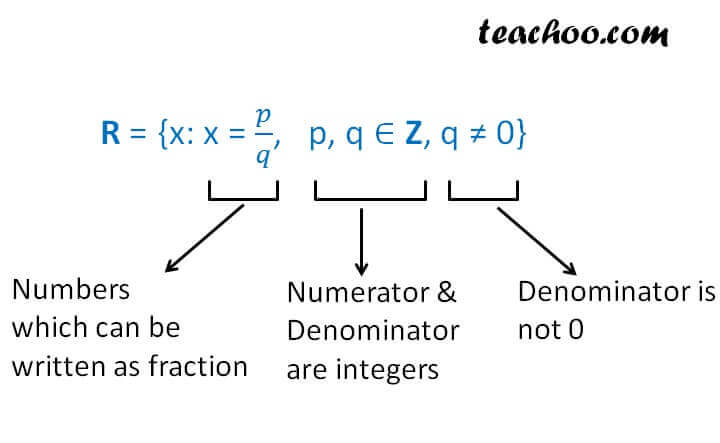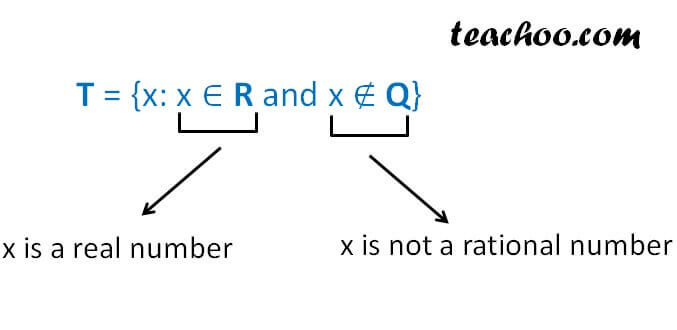Subset

Chapter 1 Class 11 Sets
Concept wise

We saw that some common sets are numbers

N : the set of all natural numbers

Z : the set of all integers

Q : the set of all rational numbers

T : the set of irrational numbers

R : the set of real numbers

Let us check all the sets one by one.

## Natural numbers

Natural numbers are numbers starting from 1.

Natural numbers = 1, 2, 3, 4, 5, …

So, N = {1, 2, 3, 4, 5, ….}

## Integers

Integers are positive numbers, negative numbers and 0.

Integers = …., -3, -2, -1, 0, 1, 2, 3, …

So, Z = {…., -3, -2, -1, 0, 1, 2, 3, …}

## Rational numbers

Rational numbers are those numbers which are of the form p/q

Example: 1/2, 2/3, …

So, we write set of rational numbers as## Irrational numbers

Irrational numbers are those numbers which are not of the form p/q

Example:  π, 1.10100100010000…

So, we write set of irrational numbers as## Real number

All numbers on number line are real numbers

It includes rational as well as irrational numbers

We write set of real numbers as  R

## Writing as Subsets

So, we can now write subset

N ⊂ Z ⊂ Q ⊂ R

Natural number is a subset of Integers

Integer is a subset of Rational numbers

And Rational numbers is a subset of Real numbers

Also, T ⊂ R

Also, Irrational numbers is a subset of Real numbers

Get live Maths 1-on-1 Classs - Class 6 to 12